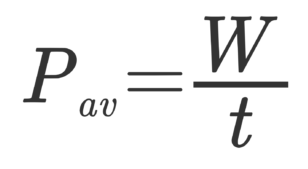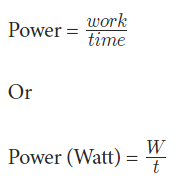# Power Formula: Derivation of Power formula, Examples

We all are very familiar with power. Also, it helps us to measure the energy that we use to do the work. In this topic, we will discuss what is power and what is Power formula, its derivation and solved examples.

## Power

Power refers to the quantity of work done by a body. Also, quantity work has to do with a force causing a displacement. Moreover, work has nothing to do with the amount of time, which this force acts to cause the displacement. In addition, there are times when we do work quickly and there are times when we do work rather slowly.For example, a trail hiker (One who selects an easier path up the mountain) can elevate her/his body a few meters in a short amount of time. On the other hand, a rock climber might take relatively more time to elevate her/his body up a few meters next to the side of a cliff.

Although, both of them do the same work but the rock climber might take more time than the trail hiker. Also, the quantity that has something to do with the rate at which a certain amount of work is done is called power. Besides, in the above case, the hiker has greater power rating than the rock climber.

### Power Formula

We can refer to power as the rate at which we do work. Also, it is the ratio of work and time. Besides, we calculate it mathematically using this formula or equation.### Derivation of Power formula

Power = unit of measure (Watt)
W = work done by the body
t = time taken to do the work

Moreover, the standard unit of measuring power is Watt. According to the power formula, a unit of power is equal to a unit of work divided by a unit of time. Also, a watt is equal to Joule/ second.

Besides, due to historical reasons, power is occasionally used to describe the power delivered by a machine. In addition, one horsepower equals 750 Watt.

Suppose that there is a car engine of 40 horsepower that could accelerate from 0 mi/hr to 60 mi/hr in 16 seconds. Moreover, if there is a car that has four times the horsepower that could do the same work in one-fourth the time. That means a 160 horsepower engine could accelerate the car from 0 mi/hr to 60 mi/hr in just 4 seconds.

Here the point is that for some amount of work, power and time are inversely proportional. Moreover, it means that a more powerful engine can do the same work in less time.

### Solved Examples on Power Formula

Example 1

Suppose that Mr. X elevates his body of 80 kg up by a 2.0-meter stairwell in 1.8 seconds. Also, in this case, then we could calculate Mr. X power rating. Besides, we must assume that Mr. X must apply an 800 Newton downward force upon the stairs to elevate his body. In addition, by doing this the stairs would push upward on Mr. X body with just enough force to lift his body up the stairs.

Besides, we can assume that the angle between the force of the stairs on Mr. X and Mr. X’s displacement is 0 degrees. Then power can be resolute as shown below:

Solution: Power = Work/Time

W = 871 Watts.

So, Mr.X power rating is 871 Watts.

Example 2

Calculate the power that a person requires to lift an object to a height of 8 m in 10 seconds. Also, the mass of the object is 10 kg. Besides, take g = 10 m/s^2

Solution: For calculating work first find the work done by the person which is equal to the potential energy at that height

Work (W) = mgh = 10 × 8 × 10 = 400J

Power =  Work/Time = 800/10 = 80 J/s.